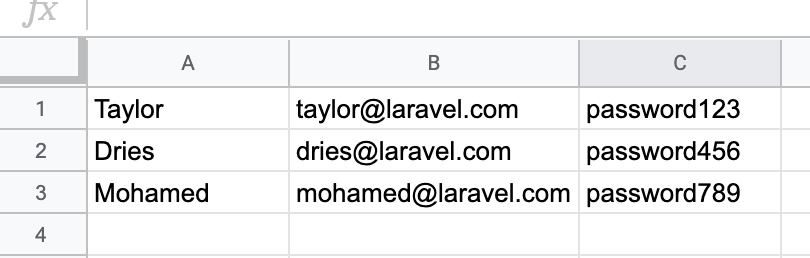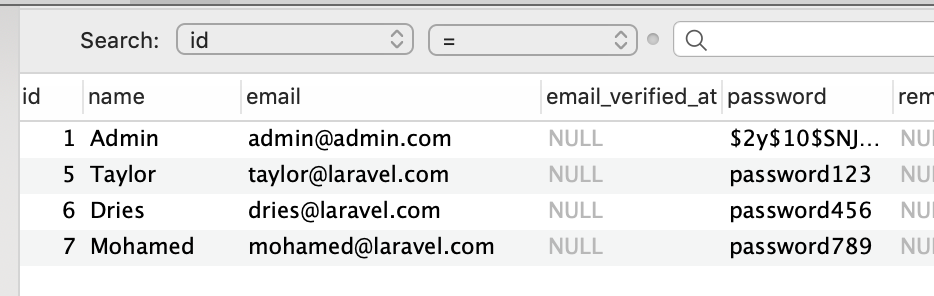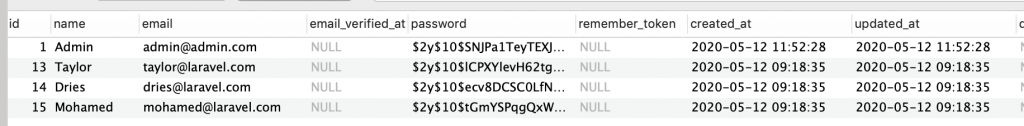Inside of QuickAdminPanel, we have a module called CSV Import. A few customers asked how to customize it, like how to handle many-to-many relationships to be imported, or modify some fields before the actual import. Let’s take a look.

## Example: Importing Default Users

Let’s try to import this CSV of users: we have only name, email, and default password.This is our default Laravel DB table users.By default, our import works without writing any custom code, just from QuickAdminPanel generated module, see the video:

But we have two problems with that:

• New users don’t get the role assigned, it should be saved in role_user pivot table
• Passwords aren’t encrypted and are saved as plain text (see below)How to customize both?

## Step 1. Copy-paste Trait into Controller

All the “magic” in CSV import is done in one file: app/Http/Controllers/Traits/CsvImportTrait.php.

And then it is included in all the Controllers where you need import. Like, app/Http/Controllers/Admin/UsersController.php:

```namespace App\Http\Controllers\Admin;

// ...
use App\Http\Controllers\Traits\CsvImportTrait;

class UsersController extends Controller
{
use CsvImportTrait;

public function index()
{
// ...
```

This is the same trait for ALL Controllers. So to make it individual for Users, we need to copy the Trait’s code into Controller. And also add a few use statements.

```// ...

use Illuminate\Support\Str;

class UsersController extends Controller
{
// ...

public function processCsvImport(Request \$request)
{
try {
\$filename = \$request->input('filename', false);
\$path     = storage_path('app/csv_import/' . \$filename);

\$fields = \$request->input('fields', false);
\$fields = array_flip(array_filter(\$fields));

\$modelName = \$request->input('modelName', false);
\$model     = "App\\" . \$modelName;

\$insert = [];

foreach (\$reader as \$key => \$row) {
if (\$hasHeader && \$key == 0) {
continue;
}

\$tmp = [];

foreach (\$fields as \$header => \$k) {
if (isset(\$row[\$k])) {
}
}

if (count(\$tmp) > 0) {
\$insert[] = \$tmp;
}
}

\$for_insert = array_chunk(\$insert, 100);

foreach (\$for_insert as \$insert_item) {
\$model::insert(\$insert_item);
}

\$rows  = count(\$insert);
\$table = Str::plural(\$modelName);

File::delete(\$path);

session()->flash('message', trans('global.app_imported_rows_to_table', ['rows' => \$rows, 'table' => \$table]));

return redirect(\$request->input("redirect"));
} catch (\Exception \$ex) {
throw \$ex;
}
}

public function parseCsvImport(Request \$request)
{
\$file = \$request->file('csv_file');
\$request->validate([
'csv_file' => 'mimes:csv,txt',
]);

\$path      = \$file->path();

\$lines   = [];

\$filename = Str::random(10) . '.csv';
\$file->storeAs('csv_import', \$filename);

\$modelName     = \$request->input('model', false);
\$fullModelName = "App\\" . \$modelName;

\$model     = new \$fullModelName();
\$fillables = \$model->getFillable();

\$redirect = url()->previous();

\$routeName = 'admin.' . strtolower(Str::plural(Str::kebab(\$modelName))) . '.processCsvImport';

}

}
```

## Step 2. Change Insert to Create and Assign Role

The main part where the record is created is here in processCsvImport() method:

```\$for_insert = array_chunk(\$insert, 100);
foreach (\$for_insert as \$insert_item) {
\$model::insert(\$insert_item);
}
```

Let’s change that to Eloquent’s create and then use many-to-many relationship to attach the role.

This is how our relationship is defined in app/User.php model:

```public function roles()
{
return \$this->belongsToMany(Role::class);
}
```

So this is our new piece in Controller:

```// We use \$insert instead of \$for_insert and not doing chunk anymore
foreach (\$insert as \$insert_item) {
\$user = \$model::create(\$insert_item);
\$user->roles()->attach(1);
}
```

## Step 3. Encrypting the password

For the password field, or for any manipulation, we can add a so-called “mutator” that would set the field’s value to the correct one.

In fact, for app/User.php model we have already set it up by default:

```public function setPasswordAttribute(\$input)
{
if (\$input) {
\$this->attributes['password'] = app('hash')->needsRehash(\$input) ? Hash::make(\$input) : \$input;
}
}
```

When we changed that ::insert() part to ::create() part, all the Eloquent “magic” is automatically turned on, including the mutators.

So if you need any kind of manipulation before saving the data, this is your way.

The final result of the import: